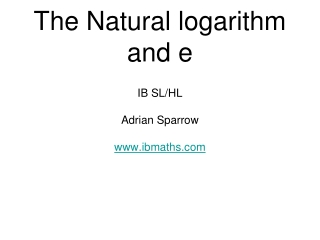DownloadDownload PresentationThe Natural logarithm and e

# The Natural logarithm and e

Télécharger la présentation## The Natural logarithm and e

- - - - - - - - - - - - - - - - - - - - - - - - - - - E N D - - - - - - - - - - - - - - - - - - - - - - - - - - -
##### Presentation Transcript

1. The Natural logarithm and e • IB SL/HL • Adrian Sparrow • www.ibmaths.com

2. Learning outcome: you should be able to solve equations by using the relationship between the natural logarithm and e.

3. Natural logarithms A logarithm to the base e is called a natural logarithm. logex is written as lnx So, lnx is the inverse function of ex

4. Without using a calculator find the value of: Using the natural log - ln Use a calculator to find: = 3 =0 = 4 =1 = =2 = -3 = = -1 = n

5. The laws of natural logarithms It is also helpful to realise that lnex= x

6. Finding a missing index using logarithms Find x to 2 decimal places using trial and error. This process can be used with any base log, even the natural log. Far too complicated ... Take a log of both sides Now try these, answers to 2 d.p. Use the power rule

7. Find x, if Find x, if Remember the base of a natural log is e. Take a natural log of both sides. Rearrange in index form. Use the power rule. Find x in each of the following: Find x in each of the following: